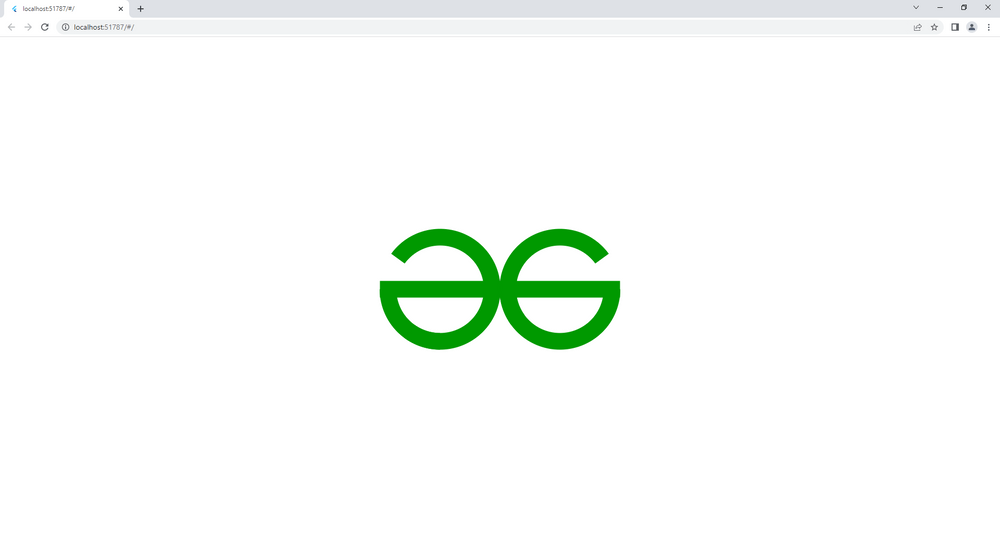Open in App
Not now

# Custom Paint Widget in Flutter

• Last Updated : 17 Jul, 2022

Flutter gives developers the full freedom to use every pixel on the screen and draw custom shapes. This has been one of the key selling points of Flutter. To achieve this, we use the CustomPainter class. In this article, we’ll try to create the GeeksforGeeks Logo using Flutter’s CustomPaint widget.

## Step by Step Implementation

Follow the below steps to draw the Geeksforgeeks logo using Custom painter in Flutter:

## Dart

 `import ``'package:flutter/material.dart'``;``import ``'dart:math'` `as math;`` ` `void` `main() {``  ``runApp(``const` `MyApp());``}`` ` `// root of our application``class` `MyApp extends StatelessWidget {``  ``const` `MyApp({Key? key}) : super(key: key);`` ` `  ``@override``  ``Widget build(BuildContext context) {``    ``return` `MaterialApp(``      ``theme: ThemeData.dark().copyWith(``        ``// the background behind the``        ``// logo should be white``        ``scaffoldBackgroundColor: Colors.white,``      ``),``      ``debugShowCheckedModeBanner: ``false``,``      ``home: ``const` `Scaffold(``        ``body: Center(``          ``// the widget that will be``          ``// responsible for displaying the logo``          ``child: MyArc(),``        ``),``      ``),``    ``);``  ``}``}`` ` `class` `MyArc extends StatelessWidget {``  ``const` `MyArc({``    ``Key? key,``  ``}) : super(key: key);`` ` `  ``@override``  ``Widget build(BuildContext context) {``    ``// for responsive design``    ``return` `LayoutBuilder(``        ``builder: (BuildContext context, BoxConstraints constraints) {``      ``// mobile screen``      ``if` `(constraints.maxWidth < 1000) {``        ``return` `const` `Logo(``          ``// the diameter is 150 is the``          ``// screen is a mobile screen``          ``diameter: 150,``          ``isMobile: ``true``,``        ``);``      ``}``      ``// desktop screen``      ``return` `const` `Logo(``        ``// the diameter is a bit larger than``        ``// the mobile screen's diameter``        ``diameter: 200,``        ``isMobile: ``false``,``      ``);``    ``});``  ``}``}`` ` `class` `Logo extends StatelessWidget {``  ``const` `Logo({``    ``Key? key,``    ``required ``this``.diameter,``    ``required ``this``.isMobile,``  ``}) : super(key: key);`` ` `  ``// the diameter of the logo``  ``final ``double` `diameter;`` ` `  ``// isMobile is true if the``  ``// screen is a mobile screen``  ``final ``bool` `isMobile;`` ` `  ``@override``  ``Widget build(BuildContext context) {``    ``// using row widget to create the``    ``// two sides of the logo``    ``// and join them after painting``    ``return` `Row(``      ``mainAxisAlignment: MainAxisAlignment.center,``      ``children: [``        ``// also has properties foregroundPainter,``        ``// which paints after drawing its children``        ``// and child, which adds widgets below this ``        ``// widget in the widget tree.``        ``// but we won't be needing these ``        ``// properties here.``        ``CustomPaint(``          ``// the left side of the logo``          ``// the painter the paints``          ``// before its children are ``          ``// drawn on the canvas``          ``painter: Left(isMobile),``          ``// providing the custom paint with the diameter``          ``size: Size(diameter, diameter),``        ``),``        ``Padding(``          ``// add some padding between the two``          ``// sides of the GFG logo``          ``// such that the two Gs just touch``          ``// each other as in the original logo``          ``padding: EdgeInsets.only(left: isMobile ? 25.0 : 30.0),``          ``child: CustomPaint(``            ``// the right side of the logo``            ``painter: Right(isMobile),``            ``size: Size(diameter, diameter),``          ``),``        ``),``      ``],``    ``);``  ``}``}`` ` `class` `Left extends CustomPainter {``  ``final ``bool` `isMobile;`` ` `  ``Left(``this``.isMobile);`` ` `  ``@override``  ``void` `paint(Canvas canvas, Size size) {``    ``Paint paint = Paint()``      ``..color = ``const` `Color(0xFF009900)``      ``// the style of the paint``      ``// default fills the shape.``      ``// but we want a stroke``      ``..style = PaintingStyle.stroke``      ``// the stroke width is a bit less in mobile screen``      ``..strokeWidth = isMobile ? 28 : 32;``    ``// draw the horizontal line in the``    ``// left side of the logo``    ``// the x offset is in negative in order``    ``// to account for the thick stroke width``    ``// the y offset is set in the middle as in``    ``// the original logo``    ``// where the horizontal line run through``    ``// the middle of the two Gs``    ``canvas.drawLine(Offset(isMobile ? -13.6 : -15.5, size.height / 2),``        ``Offset(size.width, size.height / 2), paint);`` ` `    ``// the following two blocks draw``    ``// the circles in the left side of the logo``    ``canvas.drawArc(``      ``Rect.fromCenter(``        ``center: Offset(size.height / 2, size.width / 2),``        ``height: size.height,``        ``width: size.width,``      ``),``      ``math.pi / 2,``      ``math.pi / 2,``      ``false``,``      ``paint,``    ``);``    ``canvas.drawArc(``      ``Rect.fromCenter(``        ``center: Offset(size.height / 2, size.width / 2),``        ``height: size.height,``        ``width: size.width,``      ``),``      ``-math.pi / 1.25,``      ``math.pi * 1.5,``      ``false``,``      ``paint,``    ``);``  ``}`` ` `  ``// flutter calls this method``  ``// to check if repainting is needed``  ``@override``  ``bool` `shouldRepaint(CustomPainter oldDelegate) => ``false``;``}`` ` `class` `Right extends CustomPainter {``  ``final ``bool` `isMobile;`` ` `  ``Right(``this``.isMobile);`` ` `  ``@override``  ``void` `paint(Canvas canvas, Size size) {``    ``Paint paint = Paint()``      ``..color = ``const` `Color(0xFF009900)``      ``..style = PaintingStyle.stroke``      ``..strokeWidth = isMobile ? 28 : 32;`` ` `    ``// draw the horizontal line in``    ``// the right side of the logo``    ``canvas.drawLine(``        ``Offset(``            ``isMobile ? size.width + 13.6 : size.width + 15.5, size.height / 2),``        ``Offset(0, size.height / 2),``        ``paint);``    ``canvas.drawArc(``      ``Rect.fromCenter(``        ``center: Offset(size.height / 2, size.width / 2),``        ``height: size.height,``        ``width: size.width,``      ``),``      ``math.pi / 1.25,``      ``math.pi,``      ``false``,``      ``paint,``    ``);``    ``canvas.drawArc(``      ``Rect.fromCenter(``        ``center: Offset(size.height / 2, size.width / 2),``        ``height: size.height,``        ``width: size.width,``      ``),``      ``0,``      ``math.pi,``      ``false``,``      ``paint,``    ``);``  ``}`` ` `  ``@override``  ``bool` `shouldRepaint(CustomPainter oldDelegate) => ``false``;``}`

### Step 3: Now, Hot Reload the Application to see the GeeksforGeeks Logo

Output: In this way, you can play around with the Custom Painter to create any shape you want.My Personal Notes arrow_drop_up